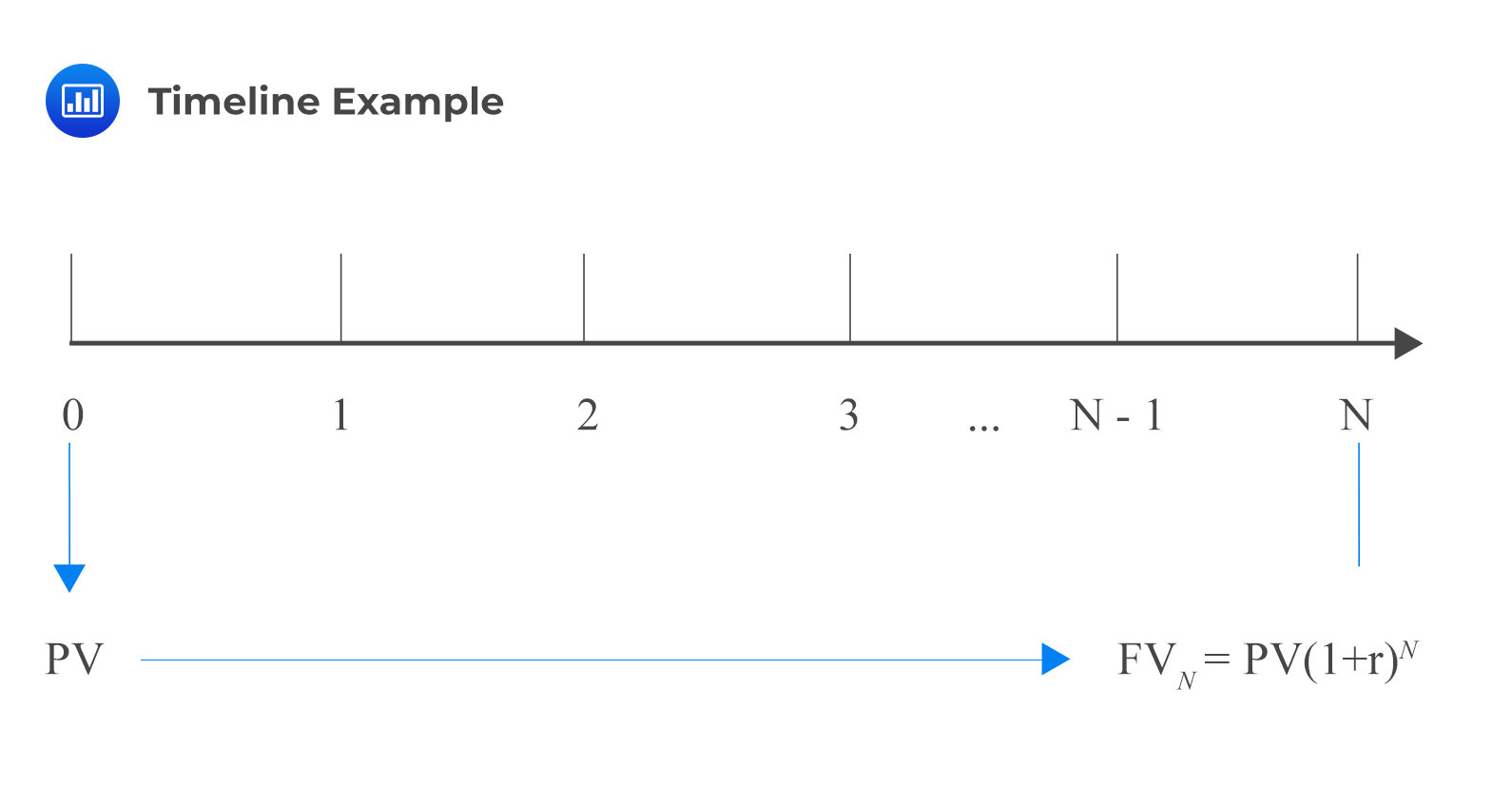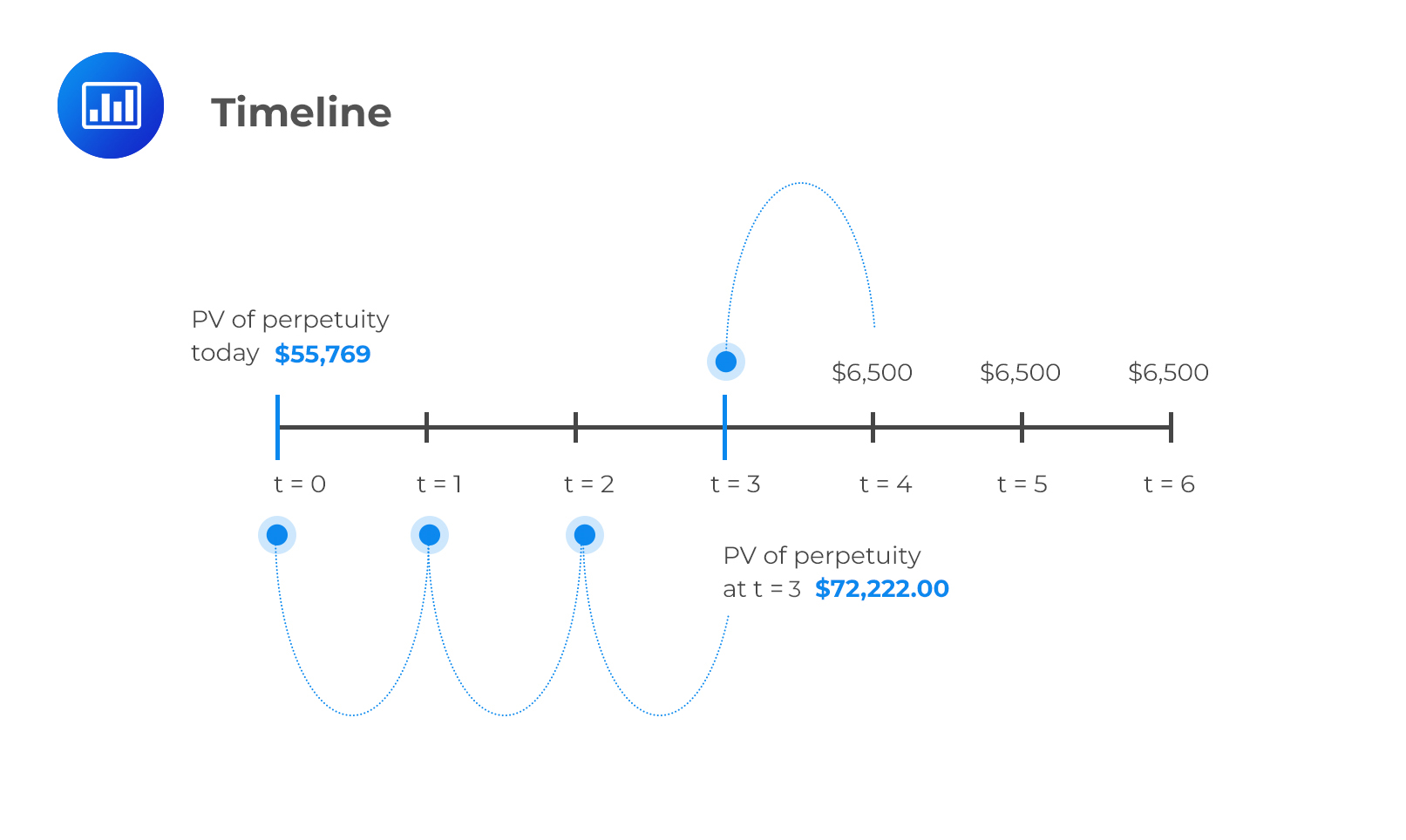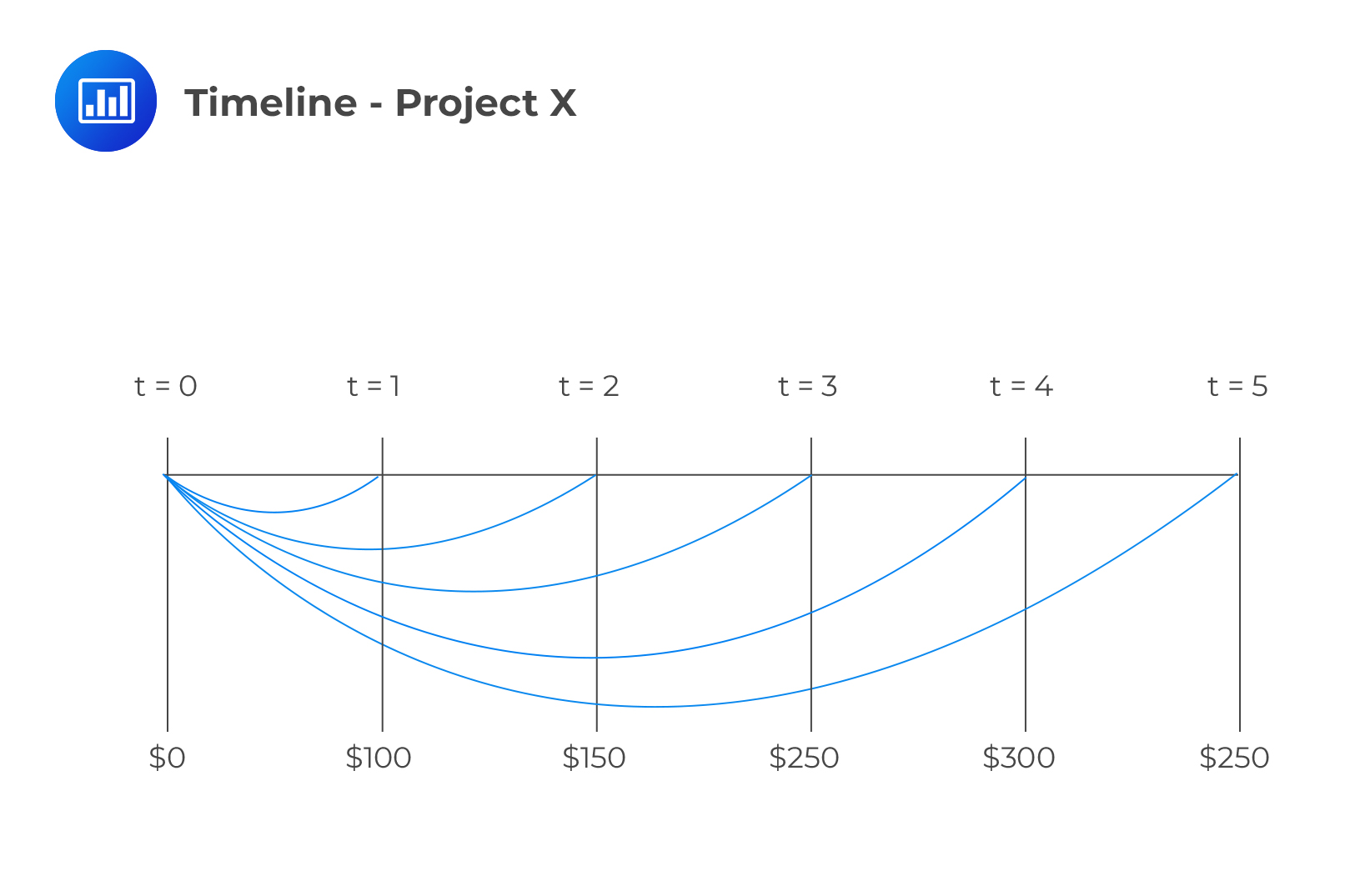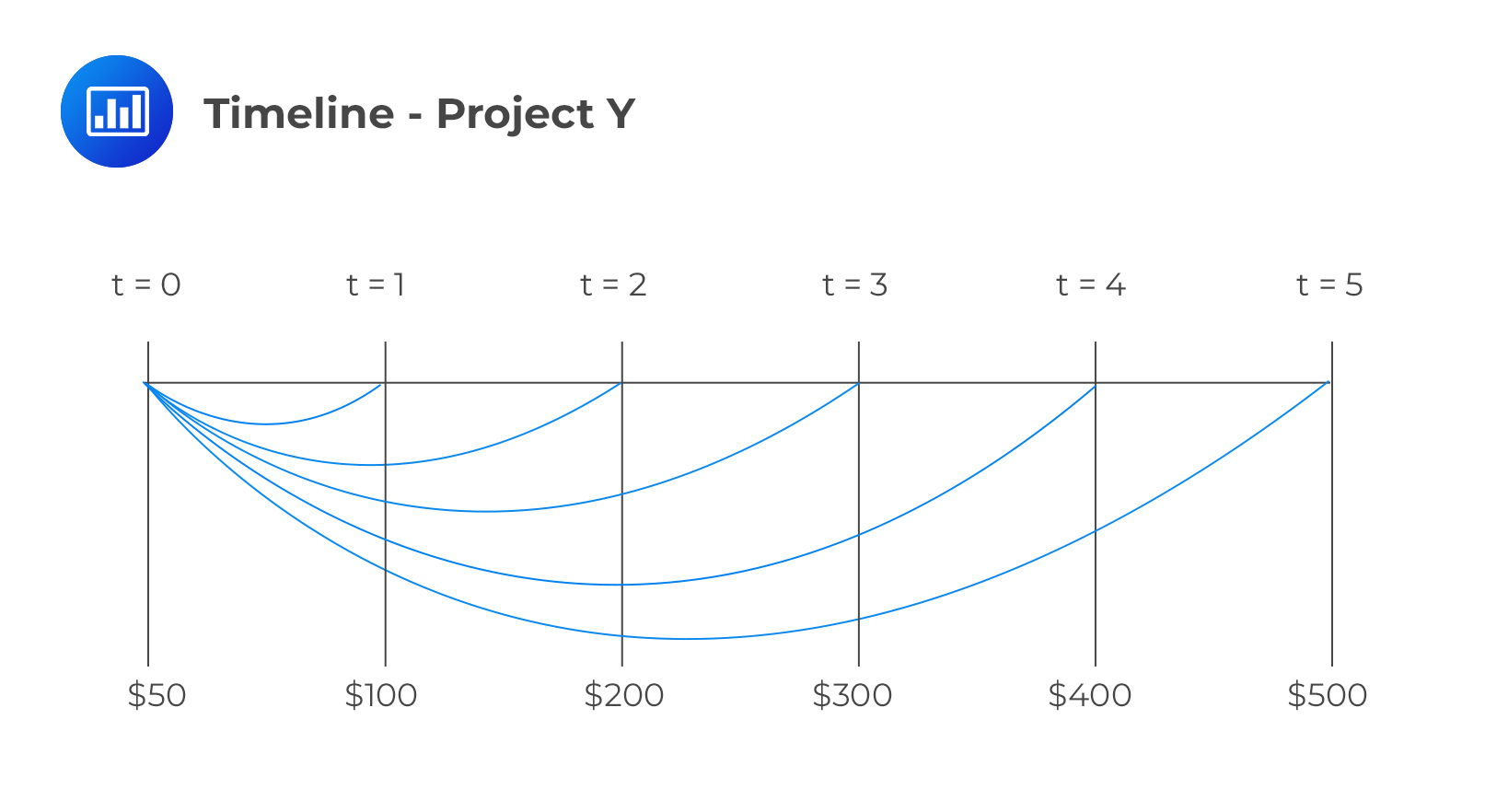# Using Timelines in Modeling and Solving Time Value of Money Problems

A timeline is a physical illustration of the amounts and timing of cash flows associated with an investment project. The standard annuity formula or the BAII Plus Pro calculator can be used for regular cashflows of equal amounts. However, a timeline is preferred for irregular, unequal, or both cashflows.

Remember that the general formula that relates the present value and the future value of an investment is given by:

$$FV _ {N} = PV \left (1 + r \right)^ {N}$$

Where:

$$PV$$ = Present value of the investment.
$$FV_N$$ = Future value of the investment $$N$$ periods from today.
$$r$$ = Rate of interest per period.

We can represent this in a timeline:In a particular timeline, a time index, t, is used to represent a particular point in time, a specified number of periods from today. Therefore, the present value is the investment amount today (t = 0), and by using this amount, we can calculate the future value (t = N). Alternatively, we can use the future value to calculate the present value.

The above argument can be written in terms of the present value. That is:

$$PV = FV _ {N} \left (1+r \right)^ {-N}$$

#### Example: Applying a Timeline to Model Cashflows

An investor receives a series of payments, each amounting to $6 500, set to be received in perpetuity. Payments are to be made at the end of each year, starting at the end of year 4. If the discount rate is 9%, then what is the present value of the perpetuity at t = 0? Solution We have to draw a timeline to understand the problem better:From the above timeline, it is indicative that the investor is receiving$6,500 in perpetuity. Remember that the PV of perpetuity is given by:

$$\text {PV of a perpetuity} = \frac {C} {r}$$

So, in this case:

$$PV_3 = \frac {6,500} {9\%} = 72, 222$$

This is the value of the perpetuity at t = 3, so we need to discount it for 3 more periods to get the value at t = 0. To do this, we use the formula:

$$PV _ 0 = FV _ {N} \left (1+r \right)^ {-N}$$

The PV at time zero is $$\frac {72, 222} {{(1 + 0.09)}^3} = 55, 769$$.

## Why is the Use of a Timeline Recommended?

There are many instances in real life when cashflows are uneven. A good example in this respect is a pension contribution which varies with age. Applying one of the basic time value formulae is impossible in such cases. It is advisable, therefore, to draw a timeline even if the question appears quite straightforward. The timeline will help you understand the question structure better. A timeline also helps candidates add cashflows indexed to the same period and apply the value additivity principle.

According to the cashflow additivity principle, the present value of any stream of cashflows indexed at the same point equals the sum of the present values of the cashflows. This principle has different applications in time value of money problems. Besides, this principle can be applied to find the NPVs of projects with uneven cash flows.

In the above question, we can combine corresponding cashflows and work out the present values for the two projects at once:

$$NPV = (0 + 50) + \frac {100 + 100} {1.05^{1}} + \frac {150 + 200} {1.05^{2}} + \frac {250 + 300} {1.05^{3}} + \frac {300 + 400} {1.05^{4}} + \frac{250 + 500} {1.05^{5}} = 2,197$$

## Calculating Interest Rates, Growth Rates, and the Number of Periods

### Calculating Interest Rate

We know that,

$$PV = FV _ {N} \left (1 + r \right)^ {-N}$$

$$\Rightarrow \text {r} = \left (\frac {FV _ N} {PV} \right)^ {\frac {1} {N}} – 1$$

#### Example: Annual Rate of Return

An investor invested in a $1,000 security today. It will earn him$1,191 three years from now. The annual rate of return on this investment is closest to?

Solution

Remember that:

$$\text {r} = \left (\frac {FV _ N} {PV} \right)^ {\frac {1} {N}} -1$$

In this case, we have:

$$\text {r} = \left (\frac {1191} {1, 000} \right)^ {\frac {1} {3}} -1 = 0.06 = 6%$$

The steps to solve the above example using a financial calculator are given below:

$$\begin {array} {l|l|l} \textbf {Steps} & \textbf {Explanation} & \textbf {Display} \\ \hline \text { [2nd] [QUIT] } & \text {Return to standard calc mode} & 0 \\ \hline\left [2^ {\text {nd }} \right] [\text {CLR TVM}] & \text {Clears TVM Worksheet} & 0 \\ \hline 3 [N] & \text {Years/periods} & N = 3 \\ \hline-1, 000 [PV] & \text {Set to } PV \text { to } – 1, 000 & PV = -1, 000 \\ \hline 0 [PMT] & \text {Set annuity payment} & PMT = 0 \\ \hline 1, 191 [FV] & \text {Set to } FV \text { to } 1, 191 & FV = 1, 191 \\ \hline [CPT] [I/Y] & \text {Compute interest rate} & I/Y = 6 \text{ or } 6 \% \\ \end{array}$$

#### Example: Annual Rate of Return #2

An investor invests $100 at the end of each of the next five years and receives$750 at the end of the fifth year. The annual rate of return on this investment is closest to:

Solution

Using a calculator, you will solve for I/Y as follows.

$$N$$ = 5; $$FV = 500$$; $$PMT = –100$$; $$CPT$$ $$I/Y$$ = 20.40%

#### Example: Annual Rate of Return #3

An investor invests $1,000 today and receives a$50 coupon payment at the end of every year for 3 years. In addition, they receive $1,100 at the end of year 3. What is the rate of return on this investment? Solution Using a calculator, you will calculate I/Y as follows. $$PV$$ = -$1, 000.

$$FV$$ = $1, 100. $$N$$ = 3. $$PMT$$ = 50. $$CPT$$ $$I/Y$$ = 8.078%. ### Calculating the Number of Periods $$N$$ can be calculated using the following formula: $$N = \frac {\left [\frac {FV} {PV} \right]} {ln (1+r)}$$ #### Example: Number of Period Calculation An investor invests$3,000 in a bank account. How many years will it take to double the amount, given that the interest rate is 5% per annum compounded annually?

Solution

Using the formula:

$$N = \frac {\left [\frac {FV} {PV} \right]} {ln(1+r)} = \left(\frac {ln 2} {ln 1.05} \right) = 14.21\ \text {Years}$$

Using the BA II Plus™ Financial Calculator:

$$I/Y$$ = 5%, $$PV$$ = -$3, 000, $$PMT$$ = 0, $$FV$$ =$6, 000, $$CPT$$ $$N$$ = 14.21

### Calculating Annuity Payments (Combining Future Value and Present Value Annuities)

The annuity payment, $$A$$, can be computed using the following formula:

\begin {align} \text {A} & = \frac {\text {PV of Annuity}} {\text {Present value annuity Factor}} \\ & = \frac {\text {PV of Annuity}} {\left[\frac {1-\frac{1} {\left(1+\frac{r s} {m}\right)^ {m N}}} {\frac {r S} {m}}\right]} \end {align}

#### Example: Annuity Payment Calculation

A company has borrowed $100,000 for the purchase of machinery. The tenor of the loan is five years at an annual rate of 5% compounded monthly. If the first payment is due in one month, the monthly payment is closest to: Solution Using the formula: $$A = \frac {\text {PV of Annuity}} {\left[\frac{1 – \frac{1} {\left(1 + \frac {r s} {m} \right)^ {m N}}} {\frac {r S}{m}} \right]} = \left [\frac {1 – \frac {1} {\left (1 + \frac {0.05} {12} \right)^ {12(5)}}} {\frac {0.05} {12}} \right] = \frac {100, 000}{52.9907} = 1, 887.1237$$ So, the monthly payment is$1,887.

Using the BA II Plus™ Financial Calculator:

$$PV$$ = -100, 000; $$N$$ = 5×12 = 60; $$I/Y$$ = 5/12 = 0.41667; $$FV$$ = 0; $$CPT \ PMT$$ = 1,887.123

A bank is offering a profit rate of 6% on its savings account. How much should an investor deposit each quarter to grow the investment to 20,000 at the end of 12 years? Solution Using the formula: \begin {aligned} F V & = A\left [\frac {\left (1+\frac {r S} {m} \right)^ {m N} -1} {\frac {r s} {m}} \right] \\ \ 20, 000 & = A\left [\frac {\left (1 + \frac {0.06} {4} \right)^ {4 \times 12} -1} {\frac {0.06} {4}}\right] \\ \ 20, 000 & = 69.5652 A \\ \Rightarrow A & = 287.50 \end{aligned} Using the BA II Plus™ Financial Calculator: $$FV$$ = -20, 000; $$N$$ = 4 × 12 = 48; $$I/Y$$ = 6/4 = 1.5; $$PV$$ = 0; $$CPT$$ $$PMT$$ = 287.50 #### Example: Annuity Payment Calculation An investor needs to accumulate3,000 over the next 5 years. How much should she invest in a savings account every year, given that the expected rate of return is 6.5%?

Solution

Using the BA II Plus™ Financial Calculator:

$$FV$$ = -3, 000; $$N$$ = 5; I/Y = 6.5; $$PV$$ = 0; $$CPT \ PMT$$ = 526.90

## Question

Assume that we have two projects, X and Y, and each has positive cashflows. The annual interest rate is 5%. The projects have the following cashflow:

Project X:

$$\begin {array} {c|c|c|c|c|c} {t = 0} & {t = 1} & {t = 2} & {t = 3} & {t = 4} & {t = 5} \\ \hline {0} & {100} & {150} & {250} & {300} & {250} \\ \end {array}$$

Project Y:

$$\begin {array} {c|c|c|c|c|c} {t=0} & {t=1} & {t = 2} & {t = 3} & {t = 4} & {t = 5} \\ \hline {50 } & {100 } & {200} & {300} & {400} & {500} \\ \end {array}$$

What is the present value of the cashflows for both projects combined?

1. $890. 2.$ 1,307.
3. \$ 2,197.

#### Solution

The timeline for project X is as follows:We can calculate the cashflows for project X as:

\begin {align*} \text {PV for X} & = 100 (1 + r)^ {-1} + 150 (1 + r)^ {-2} + 250 (1 + r)^ {-3} + 300 (1 + r)^ {-4} + 250 (1 + r)^ {-5} \\ & = 100 \times 1.05^ {-1} + 150 \times 1.05^ {-2} + 250 \times 1.05^ {-3} + 300 \times 1.05^ {-4} + 250 \times 1.05^{-5} \\ & =  890\end {align*}

For project Y, the timeline is given by:\begin {align*} \text {PV for Y} & = 50 (1 + r)^ {-0} + 100 (1 + r)^ {-1} + 200 (1 + r)^ {-2} + 300 (1 + r)^ {-3} + 400 (1 + r)^ {-4} + 500 (1 + r)^ {-5} \\ & = 50 + 100 \times 1.05^ {-1} + 200 \times 1.05^ {-2} + 300 \times 1.05^ {-3} + 400 \times 1.05^ {-4} + 500 \times1.05^ {-5} \\ & = 1307 \end {align*}

We then simply add the cashflows together:

$$PV_ {X+Y} = 890 + 1307 = 2,197$$

Shop CFA® Exam Prep

Offered by AnalystPrepLevel I
Level II
Level III
All Three Levels
Featured Shop FRM® Exam PrepFRM Part I
FRM Part II
FRM Part I & Part II
Learn with Us

Subscribe to our newsletter and keep up with the latest and greatest tips for success
Shop Actuarial Exams PrepExam P (Probability)
Exam FM (Financial Mathematics)
Exams P & FM
Shop GMAT® Exam PrepComplete CourseSergio Torrico
2021-07-23
Excelente para el FRM 2 Escribo esta revisión en español para los hispanohablantes, soy de Bolivia, y utilicé AnalystPrep para dudas y consultas sobre mi preparación para el FRM nivel 2 (lo tomé una sola vez y aprobé muy bien), siempre tuve un soporte claro, directo y rápido, el material sale rápido cuando hay cambios en el temario de GARP, y los ejercicios y exámenes son muy útiles para practicar.diana
2021-07-17
So helpful. I have been using the videos to prepare for the CFA Level II exam. The videos signpost the reading contents, explain the concepts and provide additional context for specific concepts. The fun light-hearted analogies are also a welcome break to some very dry content. I usually watch the videos before going into more in-depth reading and they are a good way to avoid being overwhelmed by the sheer volume of content when you look at the readings.Kriti Dhawan
2021-07-16
A great curriculum provider. James sir explains the concept so well that rather than memorising it, you tend to intuitively understand and absorb them. Thank you ! Grateful I saw this at the right time for my CFA prep.nikhil kumar
2021-06-28
Very well explained and gives a great insight about topics in a very short time. Glad to have found Professor Forjan's lectures.Marwan
2021-06-22
Great support throughout the course by the team, did not feel neglectedBenjamin anonymous
2021-05-10
I loved using AnalystPrep for FRM. QBank is huge, videos are great. Would recommend to a friendDaniel Glyn
2021-03-24
I have finished my FRM1 thanks to AnalystPrep. And now using AnalystPrep for my FRM2 preparation. Professor Forjan is brilliant. He gives such good explanations and analogies. And more than anything makes learning fun. A big thank you to Analystprep and Professor Forjan. 5 stars all the way!michael walshe
2021-03-18
Professor James' videos are excellent for understanding the underlying theories behind financial engineering / financial analysis. The AnalystPrep videos were better than any of the others that I searched through on YouTube for providing a clear explanation of some concepts, such as Portfolio theory, CAPM, and Arbitrage Pricing theory. Watching these cleared up many of the unclarities I had in my head. Highly recommended.